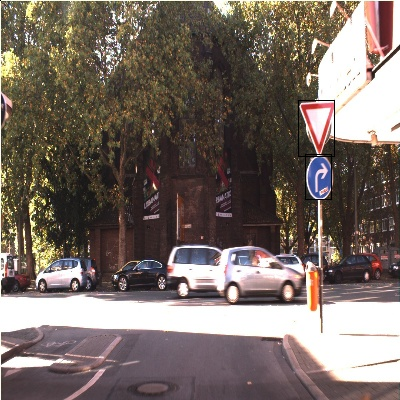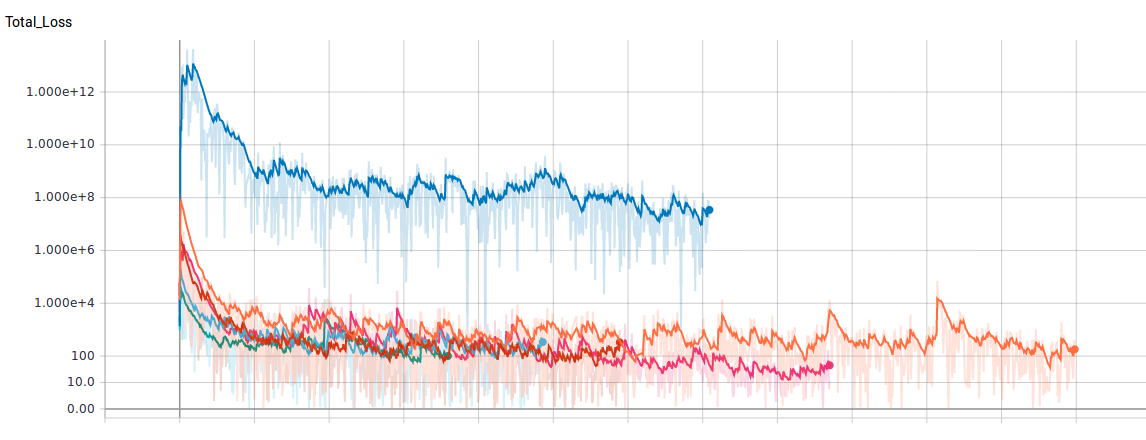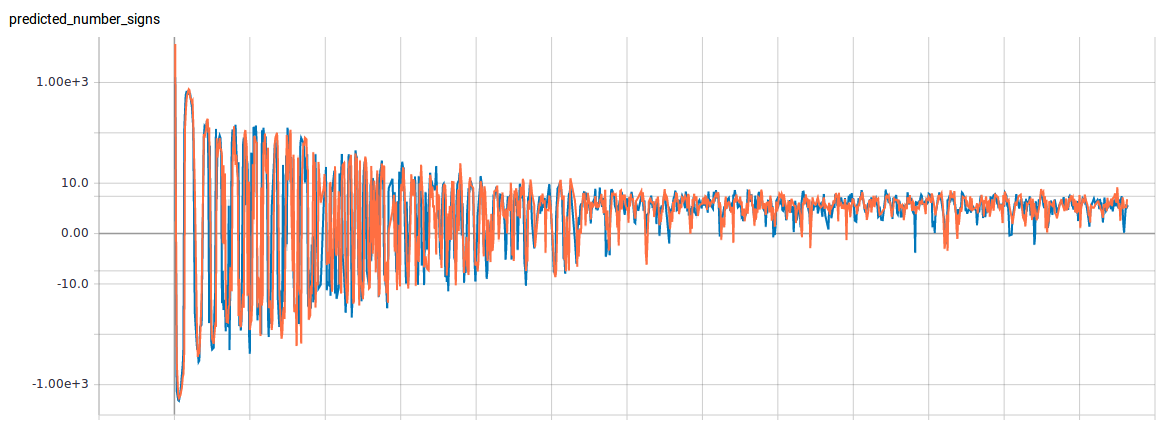Detection end-to-end

by Daniel Pollithy

The odyssey of writing a YOLO-like detection model for German traffic signs. Simply said: Give a CNN an image and let it draw boxes around the traffic signs.Getting started slowly

Before detecting concrete boxes I thought it would be a good idea to evaluate some architectures on a simpler problem. In this case I built a CNN to answer the following question:

How many traffic signs are visible in the image?

I wanted to take advantage of an ImageNet model so I took Vgg-Net (which was trained at Oxford) and used their first convolutional filters as a starter for my model.

The architecture of the CNN:

• VGG-pool-1 (all layers are freezed, trainable=False) -> batch norm
• 3 x conv with increasing filter size and stride 2,2 + batch norm
• 3 fully connected layers (the last layer outputs one single value)

Every layer uses leakyRelu except for the last FC layer.

I was using AdamOptimizer and the loss decayed pretty fast to values below 1000 but then there started an oszillation.Exactly how one would expect it to happen if the learning rate is too large and the weights “jump around” the minimum.

It was time to do a grid search on adam params.

Params are:

• learning rate
• beta1
• beta2
• epsilon

The documentation states that epsilon’s default value 1e-8 is not good. ResNet used 1.0 or 0.1!

The following was the best result after 600 iterations (which is the whole dataset). I then refined the parameters with three epochs of training per parameter combination.

lr=0.0001 b1=0.9 b2=0.8 e=1.0

And it looked good. The MSE (mean squared error) between the predicted number of signs and the ground truth went down. After 1000 iterations the MSE was below 0.5. It worked equally good on the training, test and validation data set which was surprising because usually there is a gap between results for images the network has seen before and those who are new…(blue=test, orange=train)

Intermediate status: The chosen architecture can learn a way to tell the number of traffic signs in an image so it should also be possible to find the positions of these signs.

Boxes

With the same network architecture but 30 output units I am going to train the network again. The outputs reassemble to 5 boxes each with the following parameters:

• probability of object in this box
• y1
• x1
• y2
• x2